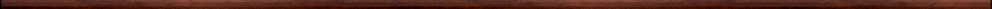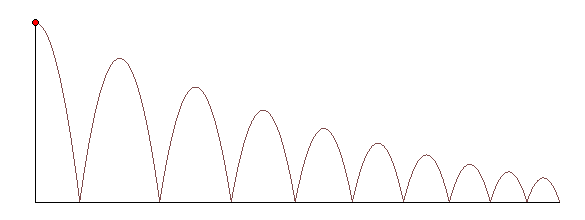Maple worksheets on sequences, series and binomial expansionsPrecalculus topics:

They are all compatible with Classic Worksheet Maple 10.An introduction to sequences including arithmetic sequences  - arithseq.mws

• Introductory examples of sequences.
• Graphs of sequences.
• Factorial notation.
• Arithmetic sequences.

An introduction to series  - series.mws

• A sum of odd numbers.
• The sum of an arithmetic series.
• Geometric sequences.
• The sum of a finite geometric series.
• A geometric series connected with compound interest on regular periodic deposits.
• The sum of an infinite geometric series.
• The distance travelled by a bouncing ball.

An introduction to counting methods  - count.mws

• The multiplication principle.
• Arrangement of n things in a line.
• A procedure for constructing arrangements of distinct items: arrange.
• General permutations of r objects taken from n.
• Using the Maple procedure combinat[permute] to list permutations.
• Selections or combinations.
• Maple procedures connected with combinations: combineperms, permstocombs, combinat[choose].

Pascal's Triangle and binomial expansions  - binomial.mws

• Expanding powers of binomials - Pascal's triangle.
• Binomial coefficients and the general binomial expansion.
• A relation between binomial coefficients.
• Constructing Pascal's triangle.

Top of page

Main index